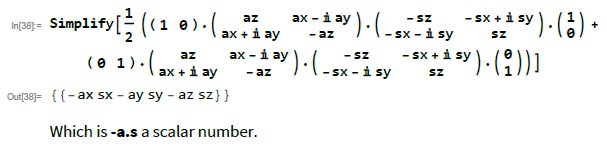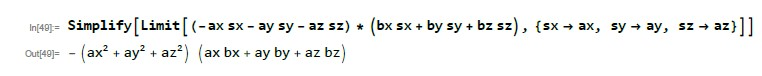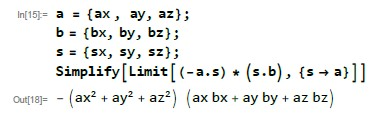## Calculations of QM prediction of -a.b

Foundations of physics and/or philosophy of physics, and in particular, posts on unresolved or controversial issues

### Re: Calculations of QM prediction of -a.b

Thank you. Please don't overlook my post about limiting both functions depending on a.
local

Posts: 220
Joined: Mon Aug 05, 2019 1:19 pm

### Re: Calculations of QM prediction of -a.b

local wrote:Thank you. Please don't overlook my post about limiting both functions depending on a.

I didn't. The Mathematica calculation is just crunching scalar numbers. There are no functions at that point.

In:= a = {ax , ay, az};
b = {bx, by, bz};
a.b

Out= ax bx + ay by + az bz

So Mathematica will do the a.b expansion going this way but will not simplify it going the other way.
.
FrediFizzx
Independent Physics Researcher

Posts: 2085
Joined: Tue Mar 19, 2013 7:12 pm
Location: N. California, USA

### Re: Calculations of QM prediction of -a.b

You haven't addressed my point. The calculation is nonlocal.
local

Posts: 220
Joined: Mon Aug 05, 2019 1:19 pm

### Re: Calculations of QM prediction of -a.b

local wrote:You haven't addressed my point. The calculation is nonlocal.

Sorry, it is not. It is like you are saying that -a.b is non-local which is pure nonsense. Once you are crunching scalar numbers, there is no locality.
.
FrediFizzx
Independent Physics Researcher

Posts: 2085
Joined: Tue Mar 19, 2013 7:12 pm
Location: N. California, USA

### Re: Calculations of QM prediction of -a.b

Here is the first part of the calculation.It is just a scalar number. Same with the other parts of the calculation.
.
FrediFizzx
Independent Physics Researcher

Posts: 2085
Joined: Tue Mar 19, 2013 7:12 pm
Location: N. California, USA

### Re: Calculations of QM prediction of -a.b

I asked why a limit operation is even necessary and pointed out that applying a limit based on a to the function for b is illegitimate. You haven't addressed either of those points. Unless you answer in a coherent way, I'm just no longer interested and don't think you have kaboshed anything.

Of course -a.b is nonlocal! It cannot be achieved with marginal (separated) sampling.

Please don't ban me for what you call nonsense. I will stay away from this thread from now on to avoid triggering you. Maybe I'll just stay away from all the threads.
local

Posts: 220
Joined: Mon Aug 05, 2019 1:19 pm

### Re: Calculations of QM prediction of -a.b

local wrote:I asked why a limit operation is even necessary and pointed out that applying a limit based on a to the function for b is illegitimate. You haven't addressed either of those points. Unless you answer in a coherent way, I'm just no longer interested and don't think you have kaboshed anything.

Of course -a.b is nonlocal! It cannot be achieved with marginal (separated) sampling.

Do you think the number -0.707 is nonlocal?-a.b could be that number. How does it have any locality to it? It is just a scalar number.
.
FrediFizzx
Independent Physics Researcher

Posts: 2085
Joined: Tue Mar 19, 2013 7:12 pm
Location: N. California, USA

### Re: Calculations of QM prediction of -a.b

Apparently the Mathematica local QM product calculation is too complicated for local to understand. So, here is a simplified version down to the last step. Hopefully local has brushed up on his vector algebra.What we have is just a product of two scalar numbers. There is no locality involved here. There are no functions here other than the limit process performed on scalar numbers.
.
FrediFizzx
Independent Physics Researcher

Posts: 2085
Joined: Tue Mar 19, 2013 7:12 pm
Location: N. California, USA

### Re: Calculations of QM prediction of -a.b

We can simplify this calculation a bit more,So, easier now to see we just have the product of two scalar numbers. Of course the Bell fans will take local's position that scalar numbers can have some kind of locality which is of course absurd.

Basically what is going on here is we are circumventing a flaw in the mathematics of quantum mechanics.
.
FrediFizzx
Independent Physics Researcher

Posts: 2085
Joined: Tue Mar 19, 2013 7:12 pm
Location: N. California, USA

### Re: Calculations of QM prediction of -a.b

So, here we have it folks. The Bell fans are now caught between a rock and a hard place. Either the local QM product calculation is correct and QM is local or local and Graft are correct that the -a.b prediction cannot be obtained from separated measurements. Fortunately we have a clue from the GA model that Nature is in fact local so I will be picking door #1. Either way, entanglement is screwed.
.
FrediFizzx
Independent Physics Researcher

Posts: 2085
Joined: Tue Mar 19, 2013 7:12 pm
Location: N. California, USA

### Re: Calculations of QM prediction of -a.b

FrediFizzx wrote:We can simplify this calculation a bit more,So, easier now to see we just have the product of two scalar numbers. Of course the Bell fans will take local's position that scalar numbers can have some kind of locality which is of course absurd.

Basically what is going on here is we are circumventing a flaw in the mathematics of quantum mechanics.
.

Fred, I don’t need Mathematica in order to show that the limit as s converges to a of (-a.s) (s.b) is - (a.a) (a.b)

You made the standard textbook QM formula for the EPR-B correlation more complicated by replacing simple things by more complicated things, and then you showed us that Mathematica can fortunately still get the same answer as before.

Are you saying that the expression with s converging to a has got some deeper physical interpretation?

FrediFizzx wrote:So, here we have it folks. The Bell fans are now caught between a rock and a hard place. Either the local QM product calculation is correct and QM is local or local and Graft are correct that the -a.b prediction cannot be obtained from separated measurements. Fortunately we have a clue from the GA model that Nature is in fact local so I will be picking door #1. Either way, entanglement is screwed.
.

The QM calculation is correct. If you want to call it “local” you can do that. Many people will agree with you. People have different understandings of what the word means, in particular, mathematicians and physicists use the word in different ways. “Local” and Graft are also correct in the sense that the -a.b prediction can’t be reproduced by local realistic models. (Graft’s model is a QM model which can be reproduced in a local realistic framework.) Lots of people find it obvious that Nature must work in a local realistic way. ie it’s an instinctual kind of knowledge. A religious conviction. It’s part of what people feel to be the fabric of reality. I guess Einstein had that feeling, Bohr somehow didn’t. It’s such a pity neither lived to comment on Bell’s work.
gill1109
Mathematical Statistician

Posts: 2051
Joined: Tue Feb 04, 2014 10:39 pm
Location: Leiden

### Re: Calculations of QM prediction of -a.b

gill1109 wrote:“Local” and Graft are also correct in the sense that the -a.b prediction can’t be reproduced by local realistic models.

SOAB, already starting up with nonsense! You know that is NOT what their assertion is. But I will repeat it again. QM can't obtain the -a.b prediction from separated measurements. It's got nothing to do with any other models. But there ARE other local models besides QM that do obtain the -a.b prediction. You know that also. So stop now talking more nonsense. First warning.
.
FrediFizzx
Independent Physics Researcher

Posts: 2085
Joined: Tue Mar 19, 2013 7:12 pm
Location: N. California, USA

### Re: Calculations of QM prediction of -a.b

By the way, neither Bohm and Aharonov (1957) nor Bell (1964) actually do the QM calculations. Bell ‘64 gives no reference to a derivation of the negative cosine but seems to assume everyone knows it. The place I learnt it from was in an expository paper by Kümmerer and Maassen (1998) https://www.worldscientific.com/doi/abs ... 16054_0003 , pay wall, preprint (1996) available here: https://dspace.library.uu.nl/handle/1874/2322

Between Bohm and Aharonov ‘57 and Bell ‘64 the QM calculations seem to have become so routine that no one bothers to write them out. And that remains so to this day, it seems. For instance, Clauser, Horne, Shimony, and Holt (1969) don’t bother to do them. The Stanford Encyclopedia of Philosophy (article on Bell’s theorem) doesn’t bother. Maybe someone here has a good reference?
gill1109
Mathematical Statistician

Posts: 2051
Joined: Tue Feb 04, 2014 10:39 pm
Location: Leiden

### Re: Calculations of QM prediction of -a.b

gill1109 wrote:Maybe someone here has a good reference?

https://www.mathpages.com/home/kmath521/kmath521.htm
Heinera

Posts: 788
Joined: Thu Feb 06, 2014 1:50 am

### Re: Calculations of QM prediction of -a.b

Heinera wrote:
gill1109 wrote:Maybe someone here has a good reference?

https://www.mathpages.com/home/kmath521/kmath521.htm

I don’t see a calculation here according to quantum mechanics for two entangled particles.
gill1109
Mathematical Statistician

Posts: 2051
Joined: Tue Feb 04, 2014 10:39 pm
Location: Leiden

### Re: Calculations of QM prediction of -a.b

gill1109 wrote:
Heinera wrote:
gill1109 wrote:Maybe someone here has a good reference?

https://www.mathpages.com/home/kmath521/kmath521.htm

I don’t see a calculation here according to quantum mechanics for two entangled particles.

The quantum mechanical calculation is trivial and you can find it in Eqs. (1) to (4) of my paper (or in Peres' book): https://arxiv.org/pdf/quant-ph/0703179.pdf.

All that is required is the rotational invariance of the singlet state to derive E(a, b) = -a.b.

***
Joy Christian
Research Physicist

Posts: 2404
Joined: Wed Feb 05, 2014 4:49 am
Location: Oxford, United Kingdom

### Re: Calculations of QM prediction of -a.b

gill1109 wrote:
Heinera wrote:
gill1109 wrote:Maybe someone here has a good reference?

https://www.mathpages.com/home/kmath521/kmath521.htm

I don’t see a calculation here according to quantum mechanics for two entangled particles.

It's embedded in the text from the paragraph starting with "These quantum mechanical predictions (which have been well supported by experiment)...".
Heinera

Posts: 788
Joined: Thu Feb 06, 2014 1:50 am

### Re: Calculations of QM prediction of -a.b

Heinera wrote:
gill1109 wrote:
Heinera wrote:
gill1109 wrote:Maybe someone here has a good reference?

https://www.mathpages.com/home/kmath521/kmath521.htm

I don’t see a calculation here according to quantum mechanics for two entangled particles.

It's embedded in the text from the paragraph starting with "These quantum mechanical predictions (which have been well supported by experiment)...".

I see! “ These quantum mechanical predictions (which have been well supported by experiment) have some remarkable implications. In the preceding example we established the initial state vector by performing a measurement in the z direction and considering only particles that yielded "spin up" in that direction. Then we subsequently performed a measurement at an angle θ relative to the positive z direction, and the probabilities of the outcomes were found to be a function of the angle θ between those two measurements. An essentially equivalent experiment can be performed by examining the spins of two spin-1/2 particles emitted in opposite directions from the decay of a singlet state with zero total spin. In such a case conservation of angular momentum requires that the spin state vectors of the individual particles are precisely opposite, so if we measure the spin of one of these particles along a certain direction and find "spin up" in that direction, then the other particle must have pure "spin down" in that direction. Thus, by measuring the spin of one particle and reducing its state vector to one of the eigenvectors of the chosen measurement basis, we automatically "collapse the wavefunction" of the other particle onto this same basis.”

One does have to carefully think for a while (indeed - one really must do a quick calculation), to confirm the assertion that the experiment claimed to be “an essentially equivalent experiment” is indeed essentially equivalent. But I agree, it is!
gill1109
Mathematical Statistician

Posts: 2051
Joined: Tue Feb 04, 2014 10:39 pm
Location: Leiden

### Re: Calculations of QM prediction of -a.b

Joy Christian wrote:
gill1109 wrote:
Heinera wrote:
gill1109 wrote:Maybe someone here has a good reference?

https://www.mathpages.com/home/kmath521/kmath521.htm

I don’t see a calculation here according to quantum mechanics for two entangled particles.

The quantum mechanical calculation is trivial and you can find it in Eqs. (1) to (4) of my paper (or in Peres' book): https://arxiv.org/pdf/quant-ph/0703179.pdf.

All that is required is the rotational invariance of the singlet state to derive E(a, b) = -a.b.

***

Thanks! You don’t do the whole calculation; you refer to Peres’ book for the hard part. But you do interestingly link it to the central GA formula. Of course the algebra generated by the three Pauli matrices is the even subalgebra of Cl(0, 3)(R), isomorphic to Cl(2, 0)(R)
gill1109
Mathematical Statistician

Posts: 2051
Joined: Tue Feb 04, 2014 10:39 pm
Location: Leiden

### Re: Calculations of QM prediction of -a.b

Leonard Susskind has a course of lectures with hyperlinks given at:
https://glenmartin.wordpress.com/home/l ... anglements

This includes nine 2-hour lectures on Quantum Entanglement and in Lecture 5 (1 hr 9 minutes into the lecture) he gets to prove that Bell's Inequalities ae broken.

As far as I remember he only breaks the inequalities and does not fill in the last step of calculating the correlation.

For theta = 45 degrees, he calculates that the classical value in the inequality is 0.125. This is the value of p++ which is in the cell corresponding to A=1 & B=1 in the 2x2 table of results for a Bell test. The correlation is easily calculated using the classical formula correl = 4*p++ -1 = -0.5.
The QM value for p++ is 0.075 and correl = 4*p++ -1 = -0.7. The QM value of p++= 0.075 breaks the Bell inequality.
Austin Fearnley

PreviousNext